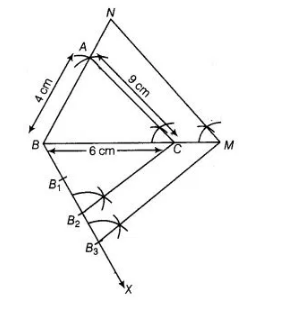# Draw a ΔABC in which AB = 4 cm,

Question:

Draw a $\triangle \mathrm{ABC}$ in which $\mathrm{AB}=4 \mathrm{~cm}, \mathrm{SC}=6 \mathrm{~cm}$ and $\mathrm{AC}=9 \mathrm{~cm}$.

Construct a triangle similar to $\triangle \mathrm{ABC}$ with scale factor $\frac{1}{4}$ Justify the construction. Are the two triangles congruent? Note

that, all the three angls and two sides of the two triangles are equal.

Solution:

Steps of construction

1. Draw a line segment BC = 6 cm.
2. Taking B and C as centres, draw two arcs of radii 4 cm and 9 cm intersecting each other at A.
3. Join BA and CA, ΔABC is the required triangle.
4. From B, draw any ray BX downwards making an acute angle.
5. Mark three points B1, B2, B3 on BX, such that BB1 = B1B2 = B2B31. Join B2C and from B3 draw B3M || B2C intersecting the extended line segment BC at
2. From point M, draw MN||CA intersecting the extended line segment BA to N.
Then,ΔNBM is the required triangle whose sides are equals to   of the corresponding sides of the ΔABC

The two triangles are not congruent because, if two triangles are congruent, then they have same shape and same size. Here, all the three angles are same but three sides are not same one side is different.

Justiflcation

Here. $B_{3} M \| B_{2} C$

$\therefore$ $\frac{B C}{C M}=\frac{2}{1}$

Now, $\frac{B M}{B C}=\frac{B C+C M}{B C}$

$=1+\frac{C M}{B C}=1+\frac{1}{2}=\frac{3}{2}$

Also, $M N \| C A$

$\therefore \quad \triangle \mathrm{ABC} \sim \triangle N B M$

Therefore, $\frac{N B}{A B}=\frac{N M}{A C}=\frac{B M}{B C}=\frac{3}{2}$